# Relative Motion in One Dimension

• Last Updated : 04 May, 2021

Mother Earth always seems to be stationary to human beings but in reality, Earth is constantly revolving around the Sun, and it is a Universal Truth. Then, why do Humans not feel the Earth moving? The answer is simple- Relative Motion, It means that the motion is always relative in Practical life. An object that is moving with a speed of V to a certain person means that the speed is measured while taking the person as a frame of reference. Similarly, when we are moving in a vehicle at a certain speed, the ground and the trees seem to be moving backwards but in reality, they are stationary and cannot move, these are real life examples and proof of the existence of Relative motion.

### Relative Motion

Relative motion is the motion compared to some frame of reference other than ground and when the motion is defined with respect to the ground, the frame of reference becomes ground. If the two objects are moving in the same direction, the relative velocity decreases for both objects. If the two objects are moving in the opposite direction, their relative velocities increase. Will there be an increase or decrease in their absolute velocities? No. Because the absolute velocity of each of them is measured w.r.t the ground (Ground is the frame of reference).

Frame of Reference:

In the simplest words, if one has to explain Frame of Reference, it would simply be the point of view, Just like humans have a different point of views, each and every other person has a different frame of reference. Imagine drawing a graph, everything is drawn with respect to origin, For instance, (4,3) is a point on the graph and while defining this pair, it is said that the point is 4 unit away on x-axis from the origin and 3 units away on y-axis from the origin, basically, everything on the graph is measured w.r.t. origin. Hence, the origin is the frame of reference here. Similarly, humans look at the world while putting them at origin and with their frame of reference.

### Relative Velocity

Suppose there are two objects P and Q moving at their respective speeds of vp and vq, now according to their positions, their relative velocities will be different. Suppose their initial displacement is X01 and X02 and their final displacement is X1 and X2 in time t when they are moving in the same direction,

Then, X1= X01 + vpt  ⇢ (1)

X2= X02+ vqt  ⇢ (2)

Subtract (1) from (2),

X2 – X1 = X01 – X02 + (vqt- vpt)

X2 – X1 = (X02 – X01) + (vq– vp)t

The final Relative displacement is given as, X = X2 – X1 (Displacement of object Q w.r.t. P)

The Initial Relative displacement is given as, X0 = X02 – X01 (displacement of object Q w.r.t. P)

The relative velocity is given as, v = vq– vp (Velocity of object Q w.r.t. object P)

### Graphs in Relative Motion

The relative velocity may Vary in value and can become positive, negative, or even zero based on the absolute values of the objects. If two velocities v1 and v2 are in same direction, the relative velocity of object 2 w.r.t 1 is (v2– v1). When these objects are moving in opposite directions, the relative velocity becomes (v2 + v1).

Let’s look at different cases based on the value of velocities (vp and vq) of a different object when the objects are moving in the same direction,

Case 1: The objects have the same velocities (vp = vq)

When the two objects have same velocity, and they are moving in the same direction, the distance between both of them will always remain constant (X2 – X1 = X)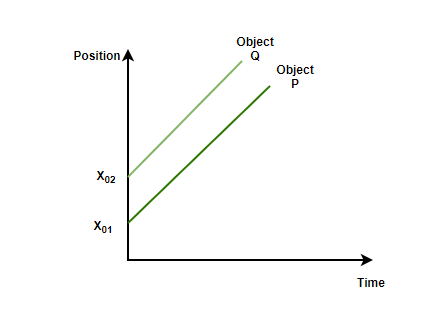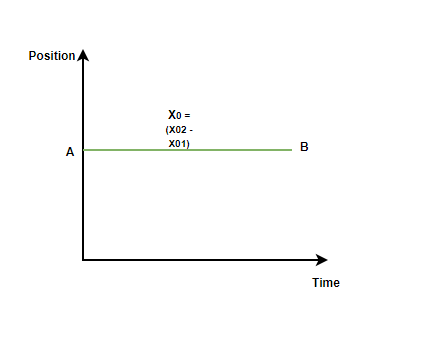Case 2: When the second object has more velocity than the first object (vq > vp)

When the second object (object Q) has more velocity than the first object (P), since Q is ahead of P, the distance between them will always be positive as P will not be able to cross Q.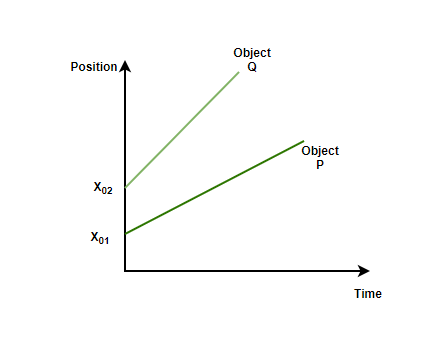Case 3: When the first object (P) has more velocity than the second object (vp > vq)

When the second object has lesser velocity, object P will be easily able to cross object Q and hence, the difference between the distance of the second object w.r.t first object will be negative.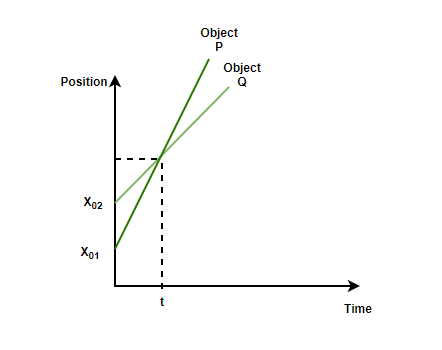Note:

• When the objects are moving in the same direction, the magnitude of the relative velocity between them is the sum of the velocities of the objects.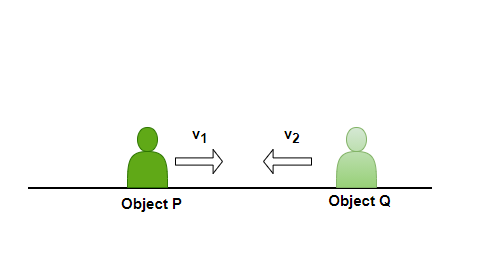• When the objects are moving in the opposite direction, the magnitude of the relative velocity between them is equal to the difference between the magnitude of their velocities.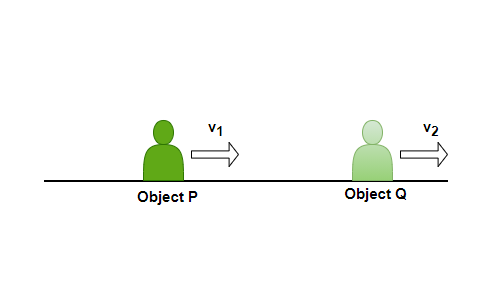### Sample Problems

Question 1: Why do the train coming in the opposite direction while travelling in trains seems to come at a very large speed?

While travelling in trains, most the times it is seen that the trains coming in opposite direction seem to be travelling at a very fast speed than usual, it is because the speed we observe is the relative speed of first train w.r.t. to the train coming in opposite direction, hence, the overall speed is the sum of speeds of both the trains.

Question 2: A particle is moving with some constant velocity of v m/sec, if it moves will the time t and covers a distance of x(t), and it is given that, x(1) = -2, x(7) = 6. Find,

1. The displacement covered by the particle from t = 1second to 7 seconds.

2. The value of the constant velocity (v m/sec).

Solution:

1. Distance at t = 1 second, x(1) = -2

Distance at t = 7 seconds, x(7) = 6

Total displacement = 6- (-2) = 6+2 = 8 units

2. Constant velocity = Displacement/ Time = 8/(7-1) = 8/6 = 4/3 m/sec.

Question 3: Rahul is riding his bicycle with a speed of 6m/sec and Raman is jogging at a speed of 2m/sec in the same direction. Prove that the relative speed of Raman w.r.t Rahul will be negative.

Solution:

Raman’s speed w.r.t. Rahul = (Speed of Raman)- (Speed of Rahul)

= 2 m/sec – 6 m/sec

= -4 m/sec

Hence, The Relative speed of Raman w.r.t. Rahul is negative.

Question 4: In the Previous Question, Hypothetically Imagine that both Rahul and Raman are on a very large platform that is moving with a speed of 10,000m/sec. Will this newly added factor have any change in the relative speed between them provided the absolute speeds to be the same as before?